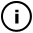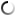# Thursday, July 23, 2009

## random Animator

int lastTime;
int currentFrame;
PImage[] frames;

boolean startedCOUNTDOWN;
int time_START;
int countdown;
int STATE;
int randomSTATE;
int randomCOUNTDOWN;

float inc_01, inc_02, inc_03, inc_04;

int decrement;

int currentSTATE;

void setup()
{
size(800, 600);
smooth();
background(255);

startedCOUNTDOWN = false;
lastTime = 0;
currentFrame = 0;
currentSTATE = 1;
frames = new PImage;

for (int i = 0; i < frames.length; i++) {
frames[i] = get(); // Create a blank frame
}

inc_01 = 0.0;
inc_02 = 0.0;
inc_03 = 0.0;
inc_04 = 0.0;
}

void draw() {
int currentTime = millis();
if (currentTime > lastTime+30) {
nextFrame();
lastTime = currentTime;
}

inc_01 = (inc_01+0.5)%60;
inc_02 = (inc_01+1)%200;
inc_03 = (inc_01+0.1)%10;
inc_04 = (inc_01+3)%99;

if (currentSTATE == 1){
countdownFunction(3);
if (mousePressed == true) {
fill(255-inc_04,50-inc_01,30-inc_02);
//noFill();
noStroke();

pushMatrix();
translate(mouseX, mouseY);
rotate(PI/inc_01);
rect(0, 0, 30-inc_01,10-inc_03);
popMatrix();
}
if(countdown == -1){
nextSTATE();
}
}
if (currentSTATE == 2){
countdownFunction(3);

if(countdown <= 3 && countdown >=0){
if (mousePressed == true) {
fill(255-inc_01,inc_03/2,90-inc_01);
noStroke();
rect(mouseX, mouseY, 30-inc_04,40-inc_02);
}
}

if(countdown == -1){
nextSTATE();
}

}

if (currentSTATE == 3){
countdownFunction(3);

if(countdown <= 3 && countdown >=0){
if (mousePressed == true) {
fill(200,100-inc_03,20-inc_04);
//noFill();
noStroke();
pushMatrix();
translate(mouseX, mouseY);
rotate(PI/inc_04);
rect(0, 0, 60-inc_04,10-inc_03);
popMatrix();
}
}

if(countdown == -1){
nextSTATE();
}

}

if (currentSTATE == 4){
countdownFunction(3);

if(countdown <= 3 && countdown >=0){
if (mousePressed == true) {
fill(150,0,20-inc_04);
noStroke();
pushMatrix();
translate(mouseX, mouseY);
rect(0, 0, 10-inc_02/3,15-inc_04);
popMatrix();

}
}

if(countdown == -1){
nextSTATE();
}

}

}

//________________GOING TO NEXT STATE____________________________
void nextSTATE(){
currentSTATE++;

startedCOUNTDOWN = false; //stop COUNTDOWN from previous screen
if (currentSTATE == 5){
currentSTATE = 1;
}
}

void nextFrame()
{
frames[currentFrame] = get(); // Get the display window
currentFrame++; // Increment to next frame
if (currentFrame >= frames.length) {
currentFrame = 0;
}
image(frames[currentFrame], 0, 0);
}

void countdownFunction (int time_TOTAL){
if(startedCOUNTDOWN == false ){

startedCOUNTDOWN = true;
time_START = millis();
}

int time_CURRENT = millis();
countdown = int(time_TOTAL - (time_CURRENT - time_START)/1000);
}

## infosubmitted by: ric_lmc
views: 2362
Click and drag the mouse to create an animation. This sketch is based on a set of looping frames on which we rewrite shapes which are slighly changing, both in their colour as well as in their shape and rotation.

Tags: animation, animator

## commentsloading...